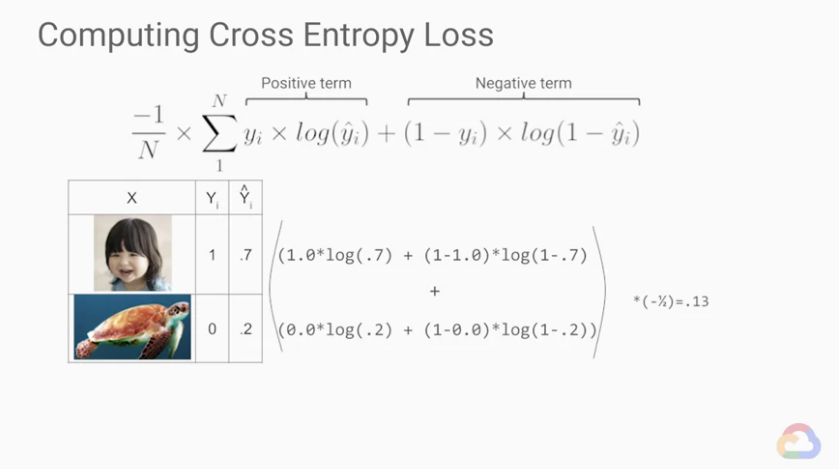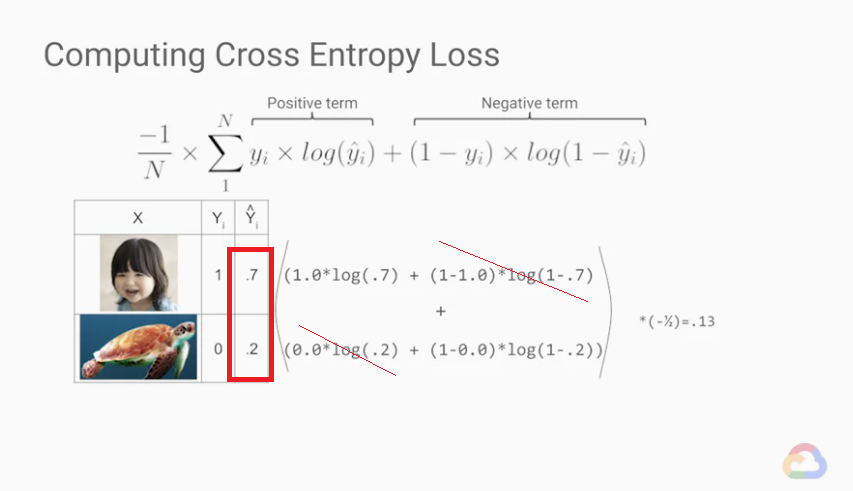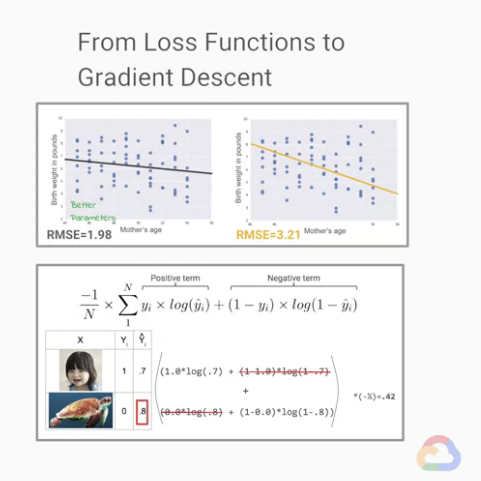DAY 20
0

# 前言

## 複習一下ML的整個訓練過程

★ML的整個「訓練過程」：這裡以`監督式學習(Supervised Learning)`為例

(`訓練前`) 決定資料集與分析資料 你想要預測的是什麼資料? 這邊需要先知道 `example``label``features`的概念。介紹可參考：【Day 15】，而我們這次作為範例的訓練資料集介紹在【Day 19】
(`訓練前`) 決定問題種類 依據資料，會知道是什麼類型的問題。`regression problem(回歸問題)`? `classification problem(分類問題)`? 此處可參考：【Day 16】、與進階內容：【Day 17】
(`訓練前`) 決定ML模型(ML models) 依據問題的種類，會知道需要使用什麼對應的ML模型。`回歸模型(Regression model)`? `分類模型(Classification model)`? 此處可參考：【Day 18】`神經網路(neural network)`? 簡介於：【Day 25】
(模型裡面的參數) ML模型裡面的`參數(parameters)``超參數(hyper-parameters)` 此處可參考：【Day 18】
(`訓練中`) 調整模型 評估當前模型好壞 `損失函數(Loss Functions)`：使用損失函數評估目前模型的好與壞。以`MSE(Mean Squared Error)`, `RMSE(Root Mean Squared Error)`, `交叉熵(Cross Entropy)`為例。此處可參考：【Day 20】
(`訓練中`) 調整模型 修正模型參數 `梯度下降法 (Gradient Descent)`為例：決定模型中參數的修正「方向」與「`步長(step size)`」此處可參考：【Day 21】
(`訓練中`) 調整腳步 調整學習腳步 透過`學習速率(learning rate)`來調整ML模型訓練的`步長(step size)`，調整學習腳步。(此參數在`訓練前`設定，為`hyper-parameter`)。此處可參考：【Day 22】
(`訓練中`) 加快訓練 取樣與分堆 設定`batch size`，透過`batch`從訓練目標中取樣，來加快ML模型訓練的速度。(此參數在`訓練前`設定，為`hyper-parameter`)。與`迭代(iteration)`,`epoch`介紹。此處可參考：【Day 23】
(`訓練中`) 加快訓練 檢查loss的頻率 調整「檢查loss的頻率」，依據`時間(Time-based)``步驟(Step-based)`。此處可參考：【Day 23】
(`訓練中`) 完成訓練 (loop) -> 完成 重覆過程(評估當前模型好壞 -> 修正模型參數)，直到能`通過「驗證資料集(Validation)」的驗證`即可結束訓練。此處可參考：【Day 27】
(`訓練後`) 訓練結果可能問題 `「不適當的最小loss?」` 此處可參考：【Day 28】
(`訓練後`) 訓練結果可能問題 `欠擬合(underfitting)`?`過度擬合(overfitting)`? 此處可參考：【Day 26】
(`訓練後`) 評估 - 性能指標 `性能指標(performance metrics)`：以`混淆矩陣(confusion matrix)`分析，包含「`Accuracy`」、「`Precision`」、「`Recall`」三種評估指標。簡介於：【Day 28】、詳細介紹於：【Day 29】
(`訓練後`) 評估 - 新資料適用性 `泛化(Generalization)`：對於新資料、沒看過的資料的模型適用性。此處可參考：【Day 26】
(`訓練後`) 評估 - 模型測試 使用「`獨立測試資料集(Test)`」測試? 使用`交叉驗證(cross-validation)`(又稱`bootstrapping`)測試? 此處可參考：【Day 27】
(資料分堆的方式) (`訓練前`) 依據上方「模型測試」的方法，決定資料分堆的方式：訓練用(Training)、驗證用(Validation)、測試用(Test)。此處可參考：【Day 27】

# Course - Launching into Machine Learning

• `Optimization`
• Introduction to Optimization
• Introduction
• Defining ML Models
• Defining ML Models
• Introducing the Natality Dataset
• `Introducing Loss Functions`
• Troubleshooting a Loss Curve
• ML Model Pitfalls
• TensorFlow Playground
• Lab: Introducing the TensorFlow Playground
• Lab: TensorFlow Playground - Advanced
• Lab: Practicing with Neural Networks
• Loss Curve Troubleshooting
• Performance Metrics
• Performance Metrics
• Confusion Matrix
• Module Quiz

## 1. Introducing Loss Functions

• Optimization
• Introducing Loss Functions

【Day 19】 中，我們曾經提過在數據集不大時，可以使用的統計方法。

### 1.1 `regression problems(回歸問題)` 的損失函數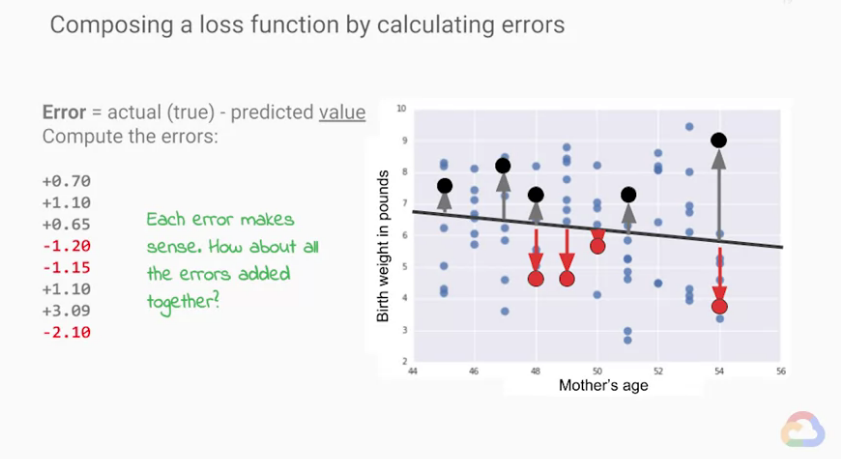• 最簡單的方法，就是直接加總，例如使用sum。

• 那「誤差(`error`)」的絕對值之和呢?

★就留個可參考的資料給有興趣的人：機器/深度學習: 基礎介紹-損失函數(loss function)

• 我們常用的方法：`MSE(Mean Squared Error)`

`MSE(Mean Squared Error)`的算法是從我們的所有數據中，

1. 拿預測的值(predictd value)與真實數據(labeled value)相減
2. 所有的相減值皆平方(避免誤差正負相消)，並取總和
3. 再除以總數量平均

MSE方法計算結果是很值得參考的，確實很適合作為我們的 loss function。

• `RMSE(Root Mean Squared Error)` 以獲得我們能解釋的單位。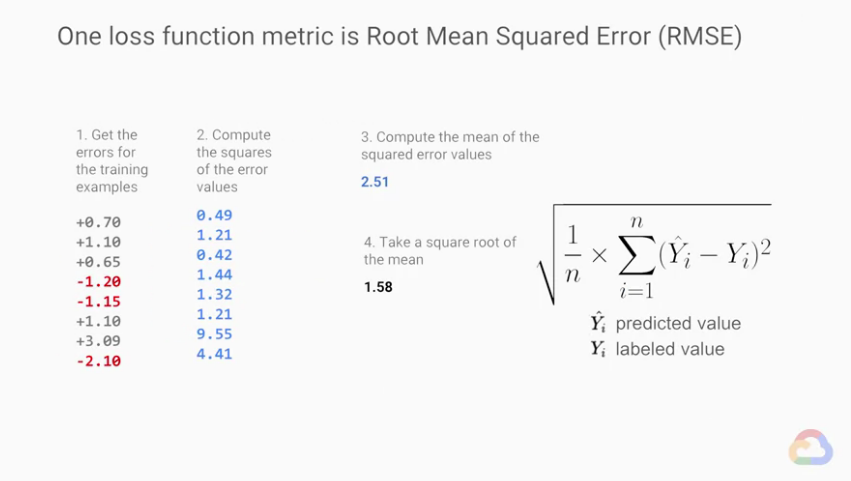(RMSE的算法，少做開根號的動作即為MSE。)
(圖中`ŷ`表示我們預測的值(predictd value)、`y`表示真實數據(`labeled value`))

`RMSE(Root Mean Squared Error)`的數值越大，同時也能表示我們預測的表現越差。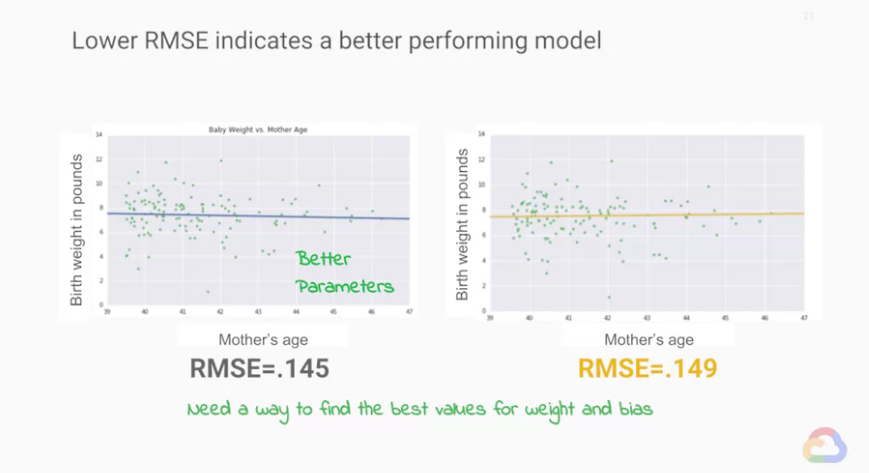「目前模型訓練的結果比較好」：表示有較好的`weight``bias`

### 1.2 `classification problems(分類問題)` 的損失函數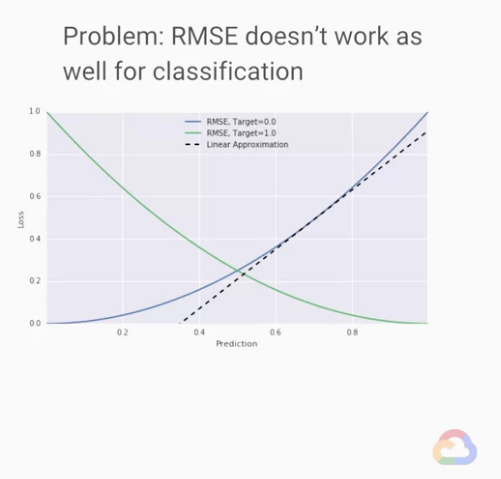(用`RMSE`預測分類問題的結果。下方內容有圖片解釋。)

`線性回歸問題(linear regression problems`)的表現很好，
`分類問題(classification problems`)似乎不行。

• X軸表示我們的預測結果(prediction)
• Y軸表示(loss)，表示預測值(prediction)與實際值(`label`)的RMSE誤差
• 藍色為預測結果是0所畫出來的線
• 綠色為預測結果是1所畫出來的線

(很多的「0.5-0」然後平方、除總數、開根)

(自己的註：「直覺的懲罰」的概念要比較下圖比較好懂，下面會解釋。)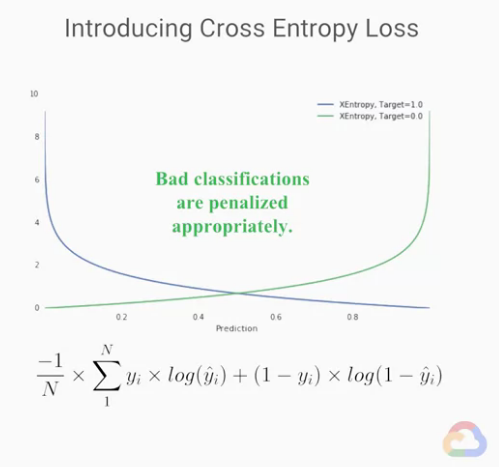`交叉熵(Cross Entropy)`是最常使用於分類問題的損失函數(loss functions)。

`交叉熵(Cross Entropy)`又有個別名`log loss`

• 我們再拿我之前【Day 17】做的表格看一下、順便複習一下吧：

★ 均方差(mean squared error) 與 交叉熵(cross-entropy) 的比較★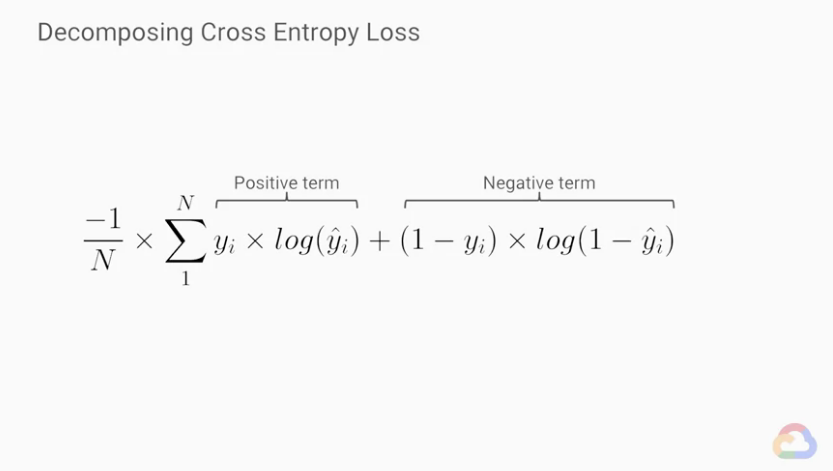• `Positive term`：當結果是1時有反應。
• `Negative term`：當結果是0時有反應。